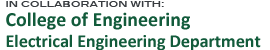Innovations in Wireless Information Networking Lab## Instructor

Richard D. Gitlin, Sc.D.
State of Florida 21st Century World Class Scholar
Distinguished Professor of Electrical Engineering

## Course Description

The course covers the basics of random processes, which is a foundation technology for many engineering domains. The course begins with a review of probability theory and functions of a random variable, joint probability distributions, and the multivariate Gaussian distribution. The course then covers the fundamentals of random processes including autocorrelation and power spectra, estimation, filtering, and prediction of random processes, and Poisson and Markov Processes.

## Topics Include

• Review of probabilty (density functions, conditional probabilty,Bayes theorem).
• Sequences of random variables.
• Introduction to random processes: stationary, cyclostationary, and ergodic.
• Autocorrelation, power spectrum, and the Wiener-Khinchin theorem.
• Spectral representations of random processes: Fourier and KarhunenLoeve series.
• Wiener, Poisson and Markov random processes.
• Linear filtering and estimation: Wiener-Hopf and Kalman filtering.
• Poisson and Markov Processes: introduction to queuing theory.
• ## Prerequisite (recommended)

EGN 3443 or equivalent first course in probability (laws of probability, Bayes' theorem, probability density function, moments) or the approval of the instructor.

## Text

Athanasios Papoulis and S. Unnikrishna Pillai, Probability, Random Variables and Stochastic Processes, Fourth Edition, McGraw-Hill, 2002, ISBN 0073660116